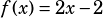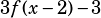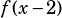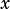Hi. Please, explain the solution to this question:

If the slope of a linear equation f(x) is 2 and the y intercept is -2, then what is the y intercept of 3f(x-2)-3

If you know the slope and the y-intercept, then you know all you need to put the f(x) into slope-intercept form (ymxb, where m is the slope and b is the y-intercept). In this case, you have.

To go from there to, your first step should be to get theout of there. To do that, just put ineverywhere the original function has:

If, then, which simplifies to.

Once you’ve got that, substitute and simplify:That’s in mxb form, so the y-intercept is –21.